# Contents

This appendix shows the relations between various piezoelectric parameters and constants and how these are affected by the mechanical and electrical boundary conditions.

Notes —

1. The following discussion uses symbols that are commonly found in the piezoelectric literature. These differ from symbols that are traditionally used for elasticity.
2.  Table G1. Piezoelectric symbols
Stress $$\sigma$$ $$T$$
Strain $$\epsilon$$ $$S$$
Young's modulus $$E$$ $$Y$$
3. The equations below typically use a single symbol to desigate a characteristic of the ceramic. Actually, however, this symbol often represents a matrix of property constants. For example, the elastic compliance is designated by $$s$$ which is actually the matrix —
4. \begin{align} \label{eq:10001b} \nonumber s = \left[ \begin{matrix} s_ {11} &s_ {12} &s_ {13} &0 &0 &0 \\ s_ {21} &s_ {22} &s_ {23} &0 &0 &0 \\ s_ {31} &s_ {32} &s_ {33} &0 &0 &0 \\ 0 &0 &0 &s_ {44} &0 &0 \\ 0 &0 &0 &0 &s_ {55} &0 \\ 0 &0 &0 &0 &0 &s_ {66} \\ \end{matrix} \right] \end{align}

To avoid some complexity associated with manipulating such matrices and without undue loss of generality, the following will assume that all stresses are uniaxial (i.e., no transverse or shear stresses) and that the piezoelectric materials are isotropic. (In fact, piezo ceramics can be quite anisotropic. For example, Piezo Technologies (p. 6) states that "the speed of sound in the radial direction is often 20% to 30% higher than the speed in the axial direction.")

5. The equations below assume linear behavior which will be true at low drive levels. However, ceramic behavior becomes nonlinear at high drive levels; none-the-less, the equations give guidance to the relationships among the parameters.

# Basic piezoelectric relations

All solids deform when subjected to an applied force. The relation between strain $$S$$ and stress $$T$$ is —

\begin{align} \label{eq:10001a} S &= s \,T \end{align}

where $$s$$ (lower case) is the material's compliance (the inverse of Young's modulus). This equation assumes a linear relation between $$S$$ and $$T$$, at least over the range of interest. This linearity will be assumed for all subsequent equations.

If a material is dielectric then the relation between the charge $$D$$ and the applied electric field $$E$$ is —

\begin{align} \label{eq:10002a} D &= \varepsilon \,E \end{align}

where $$\varepsilon$$ is the permittivity of the material. The above parameters are summarized in table G2.

 Table G2. Material parameters
Parameter Symbol Units
Stress $$T$$ Pa
Strain $$S$$ No units
Electric field strength $$E$$ volts/m
Dielectric displacement $$D$$ coulomb/m²
Compliance $$s$$ 1/Pa
Permittivity $$\varepsilon$$ (coulomb/volt)/m

Note that equations \eqref{eq:10001a} and \eqref{eq:10002a} do not involve any piezoelectric effects (i.e., there is no interaction between the mechanical effects of equation \eqref{eq:10001a} and the electrical effects of equation \eqref{eq:10002a}). However, if a material is piezoelectric then additional terms must be added to account for the piezoelectric effect. One piezoelectric effect is that applying an electric field will cause a strain in the material (this is called the inverse or converse piezoelectric effect). Thus, equation \eqref{eq:10001a} must be modified by adding the term $$d^T E$$ to account for this effect.

\begin{align} \label{eq:10003a} S &= s^E \,T + d^T \,E \end{align}

where $$d$$ is the piezoelectric charge constant (see table G3) (The superscripts, which denote constraints, are explained below).

A second piezoelectric effect is that an external stress will cause a charge to build up in the material (this is called the direct piezoelectric effect as was first investigated by the Curies). Then equation \eqref{eq:10002a} must be modified by adding the term $$d^E T$$ to account for this effect.

\begin{align} \label{eq:10004a} D &= \varepsilon^T \,E + d^E \,T \end{align}

where $$d$$ is again the piezoelectric charge constant.

 Table G3. Piezoelectric constants
Symbol Description Units
$$s^E$$ Elastic compliance $$s$$ (inverse of Young's modulus) at constant electric field $$E$$ 1/Pa = m2/N
$$\varepsilon^T$$ Permittivity $$\varepsilon$$ of the ceramic at constant stress $$T$$

Coulomb/m2

volt/m

$$d^T$$ Charge constant $$d$$ at constant stress $$T$$
strain developed per unit of applied electric field
m/volt
$$d^E$$ Charge constant $$d$$ at constant electric field $$E$$
dielectric displacement developed per unit of applied stress
Coulomb/N

Note that if $$d$$ = 0 (i.e., the material is not piezoelectric) then equations equations \eqref{eq:10003a} and  \eqref{eq:10004a} just reduce to equations \eqref{eq:10001a} and  \eqref{eq:10002a}, respectively. Hence, the piezoelectric characteristics of the material are defined by the charge constant terms $$d^T$$ and $$d^E$$.

Superscripts (constraints). A superscript on one of the constants indicates that the superscript is being held constant. For example, consider the strain parameter $$S$$ with the superscript $$E$$ (i.e., $$s^E$$) in equation G3. $$s^E$$ indicates that the electric field strength $$E$$ is held constant when the elastic compliance $$S$$ is determined (e.g., when the material is stress-strain tensile tested). If $$E$$ were not held constant then part of the strain $$S$$ would result from the piezoelectric effect of $$d^T E$$ and the measured value of $$S$$ would not be correct. (In a similar manner, the temperature is kept constant during a stress-strain tensile test so that the strain is only affected by the stress, not by any thermal expansion.) Similarly, the dielectric constant $$\varepsilon$$ in equation G4 can only be properly evaluated when the stress $$T$$ is held constant (i.e., $$\varepsilon^T$$). Otherwise, part of the dielectric displacement $$D$$ would be due to the stress $$T$$ rather than the electric field $$E$$ and the measured value of $$\varepsilon$$ would not be correct. Common constraints are summarized in table 4.

 Table G4. Constraints
Superscript Constraint Easiest method
$$T$$ Stress = constant No applied external stress
$$S$$ Strain = constant Difficult to achieve by physical restraint. See table note 1.
$$E$$ Electric field strength = constant Ceramic is short circuited (i.e., voltage = 0)
$$D$$ Dielectric displacement = constant Ceramic is open circuited (i.e., current = 0)

Table 4 notes:

1. A state of constant strain cannot be practically achieved by physically restraining the piezoelectric ceramic. However, it can be achieved by exciting at a very high frequency where the inertial effects become so large that the ceramic effectively cannot vibrate (i.e., there are no strains). (See Cady (1), pp. 328, 572.) The same situation occurs when a single degree­of­freedom spring-mass system is excited at a frequency that is very high compared to its resonant frequency — the mass will not move from its rest position.

Equation notes:

1. Equation \eqref{eq:10003a} says that the strain $$S$$ can be changed by changing the applied stress $$T$$ and/or by applying an electric field $$E$$. If the material were not piezoelectric then the electric field $$E$$ would have no effect and \eqref{eq:10003a} would reduce to \eqref{eq:10001a} — i.e.,

\begin{align} \label{eq:10005a} S &= s^E \,T \end{align}

the usual strain-stress relation. (The same result is obtained if the ceramic electrodes are short circuited so that no electric field is possible.) Thus, $$d^T E$$ is the coupled piezoelectric factor.

2. Equation \eqref{eq:10004a} says that the dielectric displacement $$D$$ (i.e., the charge that is developed across the ceramic's electroded surfaces) can be changed by changing the applied stress $$T$$ and/or by applying an electric field $$E$$. If the material were not piezoelectric then the stress $$T$$ would have no effect on the charge and \eqref{eq:10004a} would reduce to:

\begin{align} \label{eq:10006a} D &= \varepsilon^T \,E \end{align}

This is the usual relation between charge and electric field for a capacitor. (The same result is obtained if the ceramic electrodes are open circuited so that no current can flow.) Thus, $$d^E T$$ is again the coupled piezoelectric factor.
3. Equations \eqref{eq:10003a} and \eqref{eq:10004a} are actually not complete since other pameters may affect the performance. For example, the strain $$S$$ will change if the temperature of the material changes. Therefore, a term like $$\alpha H$$ should be added, where $$\alpha$$ is the coefficient of thermal expansion and $$H$$ is the temperature rise. Thus, the effect of temperature on strain is similar to to the effect of the electric field in a piezoelectric material. If the material were magnetostrictive then this effect should also be considered. The equations given here implicitly assume that these effects are not present (for example, that the material is maintained at a constant temperature) or that these effects can be ignored.
4. Although equations \eqref{eq:10003a} and \eqref{eq:10004a} are often shown in this form, the variables can easily be rearranged to give alternate equations.
5. The condition of constant electric field (constant $$E$$) is most easily achieved by short circuiting the ceramic's electroded faces so that no electric field can exist (i.e., $$E=0$$).
6. The condition of constant stress (constant $$T$$) is most easily achieved by not applying any external stress (i.e., the applied stress $$T$$ = 0).

Significance of the charge constant d. The charge constant $$d^T$$ is particularly important because it determines how much the material expands-contracts when an electric field is applied (termed the reverse piezoelectric effect for when the transducer is used as a transmitter). When the transducer is used as a receiver $$d^E$$ determines how much charge is generated as the piezoelectric material is deformed (termed the direct piezoelectric effect). (The direct effect is relatively unimportant for industrial transducers except where a separate ceramic is used in the transducer as electrical feedback to monitor the transducer's amplitude.) In all cases a large charge constant is desirable.

Although $$d^T$$ and $$d^E$$ have different units, their numerical values in the S.I. system are identical (see Berlincourt (3), equation 24a, p. 188). Hence, equations G3 and G4 are generally written without the $$T$$ and $$E$$ superscripts on d.

\begin{align} \label{eq:10007a} S &= s^E \,T + d \,E \end{align}

\begin{align} \label{eq:10008a} D &= \varepsilon^T \,E + d \,T \end{align}

As mentioned above, these equations can be rearranged to give other constants. For example, solving equation \eqref{eq:10008a} for $$E$$ and substituting into equation \eqref{eq:10007a} gives:

\begin{align} \label{eq:10009a} S &=s^{E} \,T + \frac{d}{\varepsilon^{T}}D - \frac{d^{2}}{\varepsilon^{T}} T \\[0.7em]%complex_eqn_interline_spacing &= s^{E} \,T + \frac{d}{\varepsilon^{T}}D - \frac{s^{E}}{s^{E}} \frac{d^{2}}{\varepsilon^{T}} T \nonumber \\[0.7em]%complex_eqn_interline_spacing &= s^{E}\left[1 - \frac{d^{2}}{s^{E} \varepsilon^{T}}\right] T + \frac{d}{\varepsilon^{T}} D \nonumber \end{align}

Equation \eqref{eq:10009a} can be simplified by consolidating the constant factors into two new constants — i.e.,

\begin{align} \label{eq:10010a} \kappa &=\frac{d}{\left(s^{E} \varepsilon^{T}\right)^{1/2} } \end{align}

\begin{align} \label{eq:10011a} g^{T} =\frac{d}{\varepsilon ^{T}} \end{align}

where —

 Table G5. Piezoelectric constants
Symbol Description Units
$$\kappa$$ Electromechanical coupling coefficient (see below) No units
$$g^T$$ Voltage constant $$g$$ at constant stress $$T$$ m2/Coulomb

(See Piezoelectric coupling coefficient for an alternate derivation of $$\kappa$$ and a description of its significance.)

Then \eqref{eq:10009a} becomes:

\begin{align} \label{eq:10012a} S=s^{E} (1 - \kappa^{2}) \,T + g^{T} D \end{align}

If $$D$$ is held constant (e.g., by disconnecting the electrodes (open circuit) so that no charge can flow — i.e., $$D$$ = 0) then equation \eqref{eq:10012a} becomes:

\begin{align} \label{eq:10013a} S=\left[s^{E} (1 - \kappa^{2})\right] \,T \end{align}

Equation \eqref{eq:10013a} defines the relation between the strain and stress when the ceramic's electrodes are open circuit (i.e., $$D$$ = constant). Hence, the factor $$s^E (1 - \kappa^2)$$ is the compliance at open circuit, denoted as $$s^D$$ —

\begin{align} \label{eq:10014a} s^{D} = s^E(1 - \kappa^{2}) \end{align}

Then substituting equation \eqref{eq:10014a} into equation \eqref{eq:10012a} gives:

\begin{align} \label{eq:10015a} S=s^{D} \,T + g^{T} D \end{align}

Compared to equation \eqref{eq:10003a}, the strain $$S$$ in equation \eqref{eq:10015a} is now expressed in terms of parameters $$T$$ and $$D$$ with constants $$s^D$$ and $$g^T$$. The choice of using equation \eqref{eq:10003a} versus equation \eqref{eq:10015a} is completely arbitrary (although equation \eqref{eq:10003a} is more common).

# Young's moduli

Equation \eqref{eq:10014a} shows that the piezoelectric ceramic can have two distinct compliances, $$s^E$$ and $$s^D$$. This results from the coupling of the mechanical and electrical characteristics of the ceramic.

Equation \eqref{eq:10014a} is expressed in terms of compliances. However, since Young's modulus $$Y$$ is just the inverse of the compliance $$s$$ (i.e., $$Y=s^{-1}$$ or $$Y=1/s$$ for an isotropic material), equation \eqref{eq:10014a} can be written as —

\begin{align} \label{eq:10016b} \frac{1}{Y^D} = \frac{1}{Y^E}(1 - \kappa^{2}) \end{align}

or

\begin{align} \label{eq:10016a} Y^D=\frac{Y^E}{1 - \kappa^{2}} \end{align}

where —

 $$Y^D$$ = Young's modulus at open circuit $$Y^E$$ = Young's modulus at short circuit

(As previously noted, Young's modulus is usually represented by $$E$$. However, since $$E$$ is used here for the electric field strength, Young's modulus is represented by $$Y$$ instead.)

Thus, the ceramic has two different Young's moduli, depending on the electrical boundary conditions (short circuit or open circuit). Since $$\kappa$$ is always less than 1.0, the open circuit modulus $$Y^D$$ is always greather than the short circuit modulus $$Y^E$$.

## Physical explanation

The physical explanation is as follows. (Note — The following graphs are only for illustration. The X-axis and Y-axis grids have no particular scale and cannot be directly compared between graphs.)

Consider a ceramic that is compressed by gradually applying a force up to $$F$$ (see figure G1). If the electrodes are open circuit (no conduction path) then part of the energy of compression goes toward deforming the ceramic and part goes toward establishing an electric field (i.e., a charge separation due to the ceramic's capacitance). This is the solid line AB.

Now if the compressed ceramic is short circuited then the electric field disappears. The energy of the electric field also disappears but, because this is a conservative system, that lost energy must be converted into another form of energy — in this case, additional deformation of the ceramic (the dash-dot line BC). Thus, the ceramic compresses more at short circuit than at open circuit.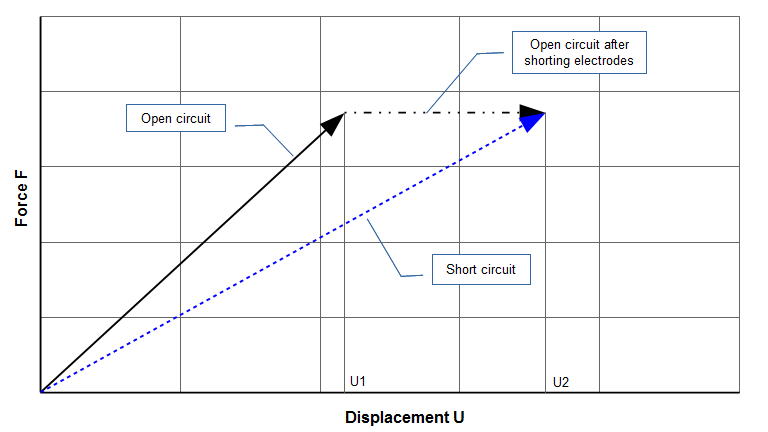Figure G1. Piezoelectric ceramic — force versus displacement

Figure G1 can be converted to the usual stress-strain diagram of figure G2. Young's modulus $$Y$$ is the slope of the appropriate stress-strain curve. Figure G1 shows that the short circuit line AC has a lower slope than the open circuit line AB (i.e., for a given stress, the strain is greater at short circuit than at open circuit). Thus, Young's modulus at short circuit ($$Y^E$$) must be lower than the Young's modulus at open circuit ($$Y^D$$).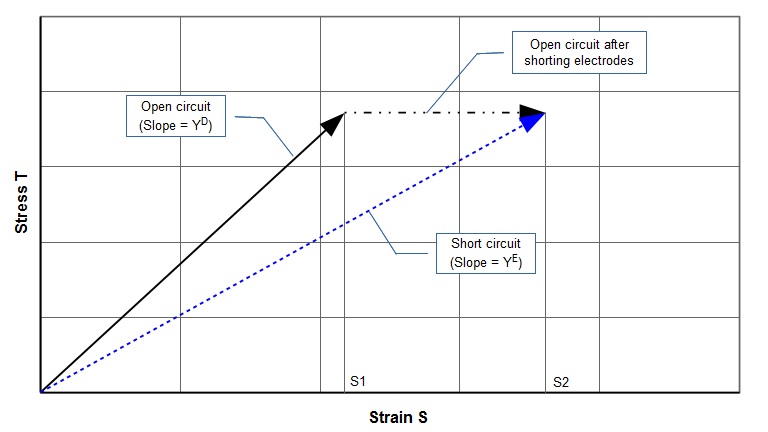Figure G2. Piezoelectric ceramic — stress versus strain

### Compressed gas analogy

Figure G3 shows a cylinder where a gas is compressed by gradually applying a force up to $$F$$. In the left image the cylinder is insulated so that no heat can escape. This is the adiabatic condition (the solid line AB in figure G4). During the compression process the temperature (thermal energy) of the gas has increased compared to its uncompressed state. The increase in thermal energy limits the displacement to $$U_1$$.

Now suppose that the excess thermal energy is allowed to drain off through the walls of the cylinder so that the temperature returns to its original state. Since the piston force is unchanged, the loss of thermal energy means that the gas can no longer support the same piston force at its previous position; thus the piston drops to a new equilibrium position $$U_2$$ (the right cylinder image). This is the dash-dot line BC in figure G4.

The same end-state C would have been achieved if the cylinder had been uninsulated during the entire loading process. Then no thermal energy would have accumulated and the loading would have progressed smoothly from A to C (the dashed line). This is the isothermal (constant temperature) condition.

Thus the gas compresses more under isothermal conditions (line AC) than under adiabatic conditions (line AB) so the isothermal bulk modulus ($$B_T$$) must be lower than the adiabatic bulk modulus ($$B_S$$). This is similar to the piezoelectric modulus ($$Y$$) where the short circuit modulus ($$Y^E$$) is lower than the open circuit modulus ($$Y^D$$).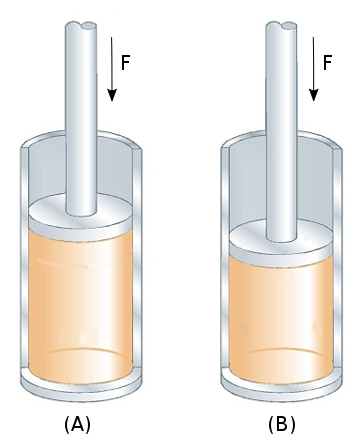Figure G3. Compressed gas analogy — (A) Adiabatic, (B) Isothermal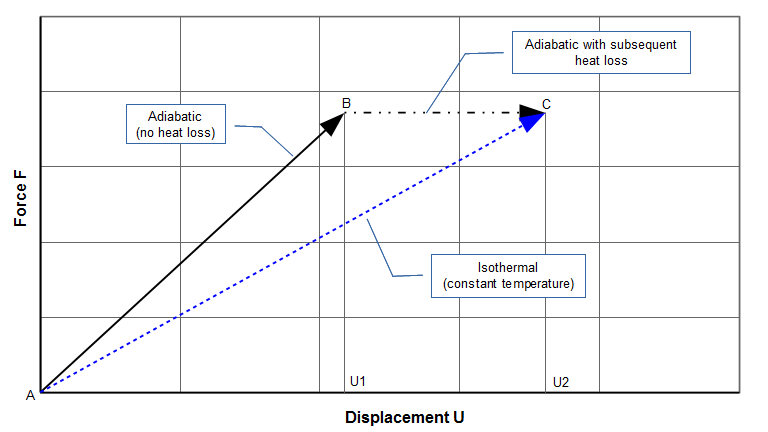Figure G4. Compressed gas — adiabatic versus isothermal

## Short circuit and open circuit resonances

Since the ceramic has two Young's moduli, it must also have two associated resonances. The resonance that is associated with $$Y^E$$ is called the short circuit resonance $$f_{sc}$$. The resonance that is associated with $$Y^D$$ is called the open circuit resonance $$f_{oc}$$. (The short circuit resonance is also called series resonance; the open circuit resonance is also called parallel resonance. The reasons will be discussed elsewhere. zzz explain distinction) The relation between these resonances is shown below.

Since the resonant frequencies are proportional to the square root of Young's modulus (i.e., $$f\propto \sqrt{Y}$$), equation \eqref{eq:10016a} can be written in terms of short circuit resonance $$f_{sc}$$ and open circuit resonance $$f_{oc}$$ as:

\begin{align} \label{eq:10018a} {f_{oc}}^2={f_{sc}}^2 \left(\frac{1}{1 - \kappa^{2}} \right) \end{align}

or

\begin{align} \label{eq:10019a} {f_{oc}}={f_{sc}} \sqrt{\frac{1}{1 - \kappa^{2}} } \end{align}

where —

 $$f_{sc}$$ = short circuit resonance $$f_{oc}$$ = open circuit resonance

Note that $$\kappa$$ has a value between 0 and 1 (see below) so the quantity under the radical of equation \eqref{eq:10019a} is always $$\geq 1$$. This means that the open circuit resonance $$f_{oc}$$ will always be greater than the short circuit resonance $$f_{sc}$$; the relative difference will depend on the coupling coefficient $$\kappa$$.

Figure G6 shows a frequency response impedance plot for the 20 kHz transducer (Branson 502) of figure G5. The impedance dip corresponds to the short circuit resonance $$f_{sc}$$. The impedance peak corresponds to the open circuit resonance $$f_{oc}$$. (This transducer is designed to operate at parallel resonance. The parallel resonance plot frequency is somewhat high because the transducer does not have a front stud. The addition of the front stud would drop the frequency into the desired operating range.)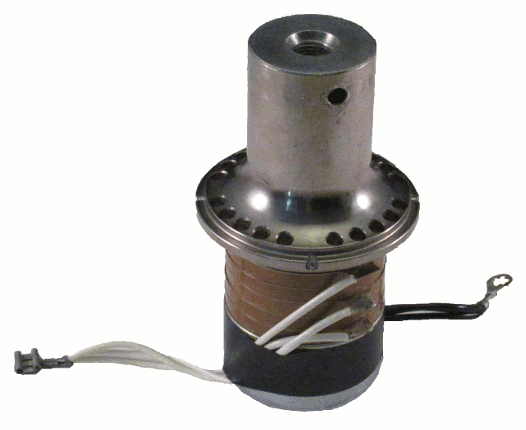Figure G5. 20 kHz industrial transducer with six piezoelectric ceramics (33 mode)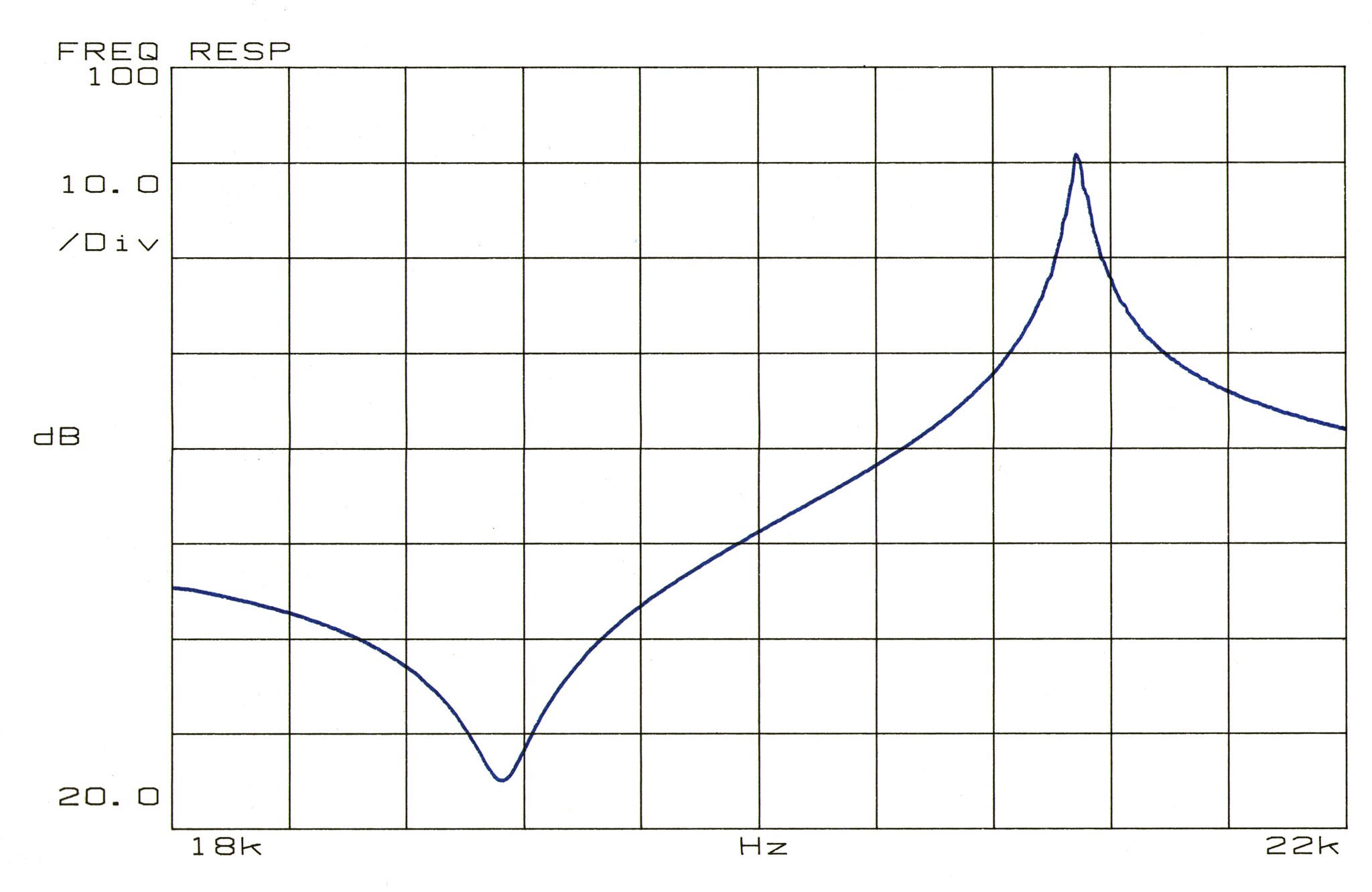Figure G6. Frequency response impedance plot for 20 kHz transducer

# Piezoelectric coupling coefficient κ

The energy that is stored in a piezoelectric material can take three forms — elastic (strain) energy, capacitive electrical energy, and coupled electromechanical energy. To show this, equations \eqref{eq:10003a} and \eqref{eq:10004a} can be expressed in terms of energies by multiplying \eqref{eq:10007a} through by $$T$$/2 and \eqref{eq:10008a} by $$E$$/2:

\begin{align} \label{eq:10020a} \small\frac{1}{2}\normalsize \,S \,T &= \small\frac{1}{2}\normalsize \,s^E \,T^2 + \small\frac{1}{2}\normalsize \,d \,E \,T \end{align}

\begin{align} \label{eq:10021a} \small\frac{1}{2}\normalsize \,D \,E &= \small\frac{1}{2}\normalsize \,\varepsilon^T \,E^2 + \small\frac{1}{2}\normalsize \,d \,E \,T \end{align}

Note that the energies in these equtions are actually energy densities (i.e., energy per unit volume).

In equation \eqref{eq:10020a}, $$\frac{1}{2} S \, T$$ is the total stored mechanical energy. This is composed of the strain energy $$\widehat{W}_1$$ ($$= \frac{1}{2} s^E \, T^2$$) and the coupled piezoelectric energy $$\widehat{W}_{12}$$ ($$= \frac{1}{2} d \,E \, T$$).

In equation \eqref{eq:10021a}, $$\frac{1}{2} D \, E$$ is the total stored electrical energy. This is composed of the stored capacitive energy $$\widehat{W}_2$$ ($$= \frac{1}{2} \varepsilon^T \, E^2$$) and, again, the coupled piezoelectric energy $$\widehat{W}_{12}$$ ($$= \frac{1}{2} d \,E \, T$$).

The coupling coefficient can be expressed in terms of the above energies as (Waanders (1), equation A8, p. 84 or Berlincourt (3), equation 30, p. 190) —

\begin{align} \label{eq:10022a} \kappa &= \frac{\widehat{W}_{12}}{(\widehat{W}_1 \,\widehat{W}_2)^{1/2}} \end{align}

where —

 $$\widehat{W}_1$$ = Total stored mechanical energy $$\widehat{W}_2$$ = Total stored electrical energy $$\widehat{W}_{12}$$ = Coupled piezoelectric energy

Substituting the specific energy terms from equations \eqref{eq:10020a} and \eqref{eq:10021a} gives —

\begin{align} \label{eq:10023a} \kappa &= \frac{\frac{1}{2} \,d \,E \,T}{\left[(\frac{1}{2} \,s^E \,T^2) \,(\frac{1}{2} \,\varepsilon^T \,E^2)\right] ^{1/2}} \\[0.7em]%complex_eqn_interline_spacing &=\frac{d}{\left(s^{E} \, \varepsilon^{T}\right)^{1/2} } \nonumber \end{align}

Note that equation \eqref{eq:10023a}, which has been derived from energy considerations, is the same as equation \eqref{eq:10010a}.

For a single piezoelectric element, $$\kappa$$ depends on the element's shape, mode of excitation (e.g., longitudinal, radial, thickness) and boundary conditions. Table G6 shows the most common conditions under which $$\kappa$$ is evaluated for single piezoelectric elements.

 Table G6. Electromechanical coupling coefficient $$\kappa$$ for single piezoelectric ceramics
Coupling coefficient Shape Electrodes Polarizing direction Vibration direction
$$\kappa_{33}$$ Long thin bar On ends Along length Along length
$$\kappa_{31}$$ Long thin bar On a pair of side faces Across thickness Along length
$$\kappa_{t}$$ Thin solid disc On circular faces Across thickness Thickness
$$\kappa_{p}$$ Thin solid disc On circular faces Across thickness Radial
$$\kappa_{u}$$ Thin ring On cylindrical faces Across cylindrical faces Radial

The above defined coupling coefficients can be used when specifying or evaluating the performance of individual ceramics. They can also be used as reference values for comparison to completely assembled transducers.

The definition of equation \eqref{eq:10019a} can be applied to a ceramic of arbitrary condition (arbitrary shape, constraints, etc.) or to an entire transducer. For these cases, however, the terminology then becomes the effective piezoelectric coupling coefficient $$\kappa_{eff}$$. (Alternately, $$\kappa_{33}$$, $$\kappa_{31}$$, $$\kappa_{t}$$, $$\kappa_{p}$$, and $$\kappa_{u}$$ can be considered special cases of the more general $$\kappa_{eff}$$.) Woollett (1) (p. 24) notes, "However,the transducer coupling coefficient is usually less than the coefficient of the material used in its construction, and is designated as the effective $$\kappa$$ to distinguish it from the $$\kappa$$ of the material." The effective value of $$\kappa$$ (i.e., $$\kappa_{eff}$$) will depend on the particular transducer's design (e.g., the effect of the stack bolt, the placement and length of the ceramics, etc.).

Any of the coupling coefficients can be calculated from their associated parallel resonance $$f_p$$ and series resonance $$f_s$$ frequencies (Waanders (1), equation A19a, p. 85).

\begin{align} \label{eq:10024a} \kappa _{eff} &= \left[\frac{{f_p}^2 - {f_s}^2}{{f_p}^2} \right]^{1/2 } \\[0.7em]%complex_eqn_interline_spacing &= \left[1 - \left({f_s}/{f_p}\right)^2 \right]^{1/2} \nonumber \end{align}

## Relation to loss

Since the parallel and series resonant frequencies are essentially independent of the transducer's mechanical and electrical losses (assuming that these are within reason), $$\kappa_{eff}$$ can't be used as a criterion for the transducer's loss. For example, for a Branson 20 kHz 502/932R transducer (figure G5) with ceramics of either moderate quality or high quality (Prokic (1), pp. 2­2, 2­3), the following table shows that $$\kappa_{eff}$$ is essentially the same for both.

 Table G7. Effect of transducer loss on $$\kappa_{eff}$$
Resonance type Parameter Moderate quality
ceramics
High quality
ceramics
Series Frequency $$f_s$$ (Hz) 18900 18815
Resistance (Ω) 4.6 1.9
$$Q$$ 454 1139
Parallel Frequency $$f_p$$ (Hz) 20912 20828
Resistance (Ω) 94 k 216 k
$$Q$$ 1257 2485
$$\kappa_{eff}$$
(equation \eqref{eq:10024a})
0.428 0.429

Note — in­so­far as loss is concerned, a higher quality transducer will be characterized by —

1. Lower resistance at series resonance
2. Higher resistance at parallel resonance
3. Higher $$Q$$

## Significance of κ

Berlincourt (3), (p. 189) asserts, "The most important properties of piezoelectric materials are their piezoelectric coupling factors."

The significance of $$\kappa$$ can be determined from equation \eqref{eq:10022a}. The numerator represents the converted energy while the denominator represents the total input energy (Waanders (1), p. 12).

\begin{align} \label{eq:10025a} \kappa &= {\left[\frac{\text{Energy converted}}{\text{Energy input}} \right]}^{1/2}_{\text {Low frequency}} \end{align}

Since the "Energy converted" must always be less than the "Energy input", $$\kappa$$ must always be less than 1.0.

In order to achieve the maximum piezoelectric effect, it is desireable to convert as much input energy into piezoelectric energy as possible. Therefore, $$\kappa$$ for the individual ceramics and $$\kappa_{eff}$$ for an assembled transducer should be as large as possible. Maximizing $$\kappa$$ will also maximize both $$d$$ and power.

### Maximized $$d$$

See equation \eqref{eq:10023a}. This will maximize the output amplitude.

### Maximized power

Berlincourt (3) (p. 250, equation 147) gives the following equation for the theoretical power that can be developed by an ultrasonic transducer. Note that the equation itself doesn't impose any limits on the parameters, particularly the electric field strength $$E$$. Practically, $$E$$ must be limited in order to prevent arcing across the ceramic's faces or to other surfaces and to prevent damage to the ceramic (depending on the type of ceramic).

\begin{align} \label{eq:10026a} p &= 2 \pi f_s \, {E_3}^2 \, \kappa^2 \, \varepsilon_{33}^T \, Q_M \end{align}

where —

 $$p$$ = Acoustic power delivered per unit volume of ceramic [W/m3] $$f_s$$ = Series resonance frequency [Hz] $$E_3$$ = Electric field strength [V/m] $$\kappa$$ = Electromechanical coupling factor [no units] $$\varepsilon_{33}^T$$ = Piezoelectric permittivity at constant stress [(coulomb/volt)/m = Farad/m] $$Q_M$$ = Mechanical Q [no units]

(Also see Woollett (1) (figure 4, p. 26).)

Thus, equation \eqref{eq:10026a} indicates that the acoustic power varies with the square of the electromechanical coupling factor. This equation is based on two assumptions —

1. The transducer can be represented as a lumped-parameter system (e.g., per Berlincourt's heavily mass-loaded transducer). In fact, practical transducers do not conform to this ideal since significant strain extends into the end masses. In that case $$\kappa$$ should be replaced by $$\kappa_{eff}$$.
2. The transducer's response is linear (e.g., with the electric field strength $$E$$). However, this assumption would likely be violated high drive levels (Woollett (1), p. 24). For example, see the following figure from Berlincourt (2). The assumption of linearity will be further disrupted when the ceramics are operated at an elevated temperature, typically due to internal heat generation.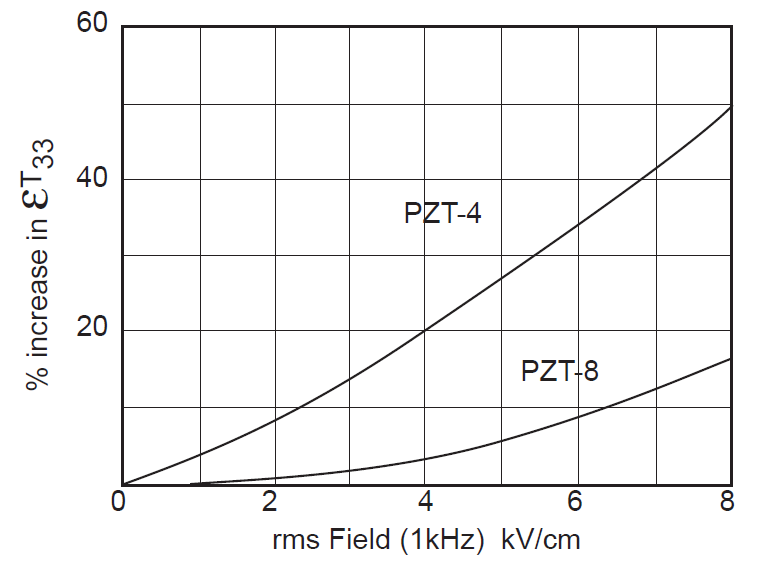Figure G7. Effect of electric field strength on piezoceramic permittivity
Thus, Woollett (1) (p. 24) notes that the predicted power will be "quite approximate" for practical transducers.

### Use for quality control

The effective electromechanical coupling coefficient can be used for partial qualification of individual ceramics — any ceramic that falls outside an acceptable range is rejected. ($$\kappa_{eff}$$ should normally be supplied by the ceramic's manufacturer. Note, however, that the manufacturer may simply designate this as electromechanical coupling coefficient or $$\kappa$$, omitting the "effective".)

Similarly, $$\kappa_{eff}$$ can be used to qualify an entire transducer. If a transducer has a $$f_s$$ that is unusually close to $$f_p$$ so that $$\kappa_{eff}$$ is unusually small (equation \eqref{eq:10024a}) then this likely indicates a defective transducer. (In this case $$\kappa_{eff}$$ of the selected transducer is compared to an average $$\kappa_{eff}$$ for known "good" transducers.)

### Improper use — comparing transducer designs

Different transducer designs can't be compared on the basis of their $$\kappa_{eff}$$.

Consider the situation where the transducer is modified so that it has more stored energy (e.g., by increasing the density of the front or back drivers, or by machining gain into the front driver). Then the difference between $$f_p$$ and $$f_s$$ will decrease (which will reduce $$\kappa_{eff}$$) even though the power handling will not be affected.

Thus, $$\kappa_{eff}$$ must be carefully interpreted. As Waanders (p. 82) notes, "Depending on the [transducer's] construction different values of $$\kappa_{eff}$$ will be found. The translation to the absolute quality level is often very complicated or impossible."

Also note that $$\kappa_{eff}$$ does not account for any transducer loss. Hence, two transducers could have identical $$\kappa_{eff}$$ yet have vastly different loss.

Given the above considerations, $$\kappa_{eff}$$ may be only marginally beneficial in comparing transducers of different designs.

## Determining κeff

$$\kappa_{eff}$$ can be determined from equation \eqref{eq:10024a} if $$f_s$$ and $$f_p$$ can be determined. This is easy and accurate for a physical transducer. The result will only be approximate otherwise. Even with FEA that can simulate the electromechanical properties of the ceramics, these properties can only be approximately estimated. For example, the Young's moduli depend on the static preload. This relationship may not be known precisely even when the prestress is uniform across the ceramic area; this is further complicated because in many cases this prestress is not uniform. Other properties present similar difficullties.